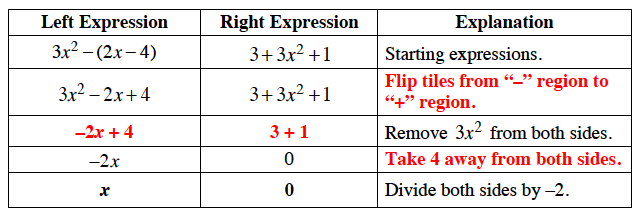### Home > CC3 > Chapter 3 > Lesson 3.1.7 > Problem3-68

3-68.

Paris is trying to solve the equation $3x^2−(2x−4)=3+3x^2+1$. Her work is partially recorded below. Copy her table and fill in her missing work to solve for $x$. Homework Help ✎

 Left Expression Right Expression Explanation $3x^2-(2x-4)$ $3+3x^2+1$ Starting expressions. $3x^2-2x+4$ $3+3x^2+1$ Remove $3x^2$ from both sides. $-2x$ $0$ Divide both sides by $-2$.

To figure out what comes next in the equation, notice what changes in each step and make sure to